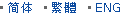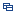| Recent Visit History | Join Audited Suppliers | Help | Region Guide
» » » » Nanjing Product List

301.

## Double Convex Lens Optical Glass Bi Convex Lens[Nov 20, 2020]

Bi Convex lens has a positive focal length, and with two outward surfaces. The two surfaces are with same radius of curvature, thereby minimizing spherical aberration. Some ...

302.

## N-Bk7 Glass Double Convex Lens for Focusing[Nov 20, 2020]

Double convex lenses comprise two convex spherical surfaces, generally with the same radius of curvature. Bi convex lenses are used as magnifiers, condensing lenses and ...

303.

## Optical Glass Double Convex Lens with Ar Coating[Nov 20, 2020]

Double Convex lens has a positive focal length, and with two outward surfaces. The two surfaces are with same radius of curvature, thereby minimizing spherical aberration. Some ...

304.

## Optical Biconvex Lens with Silicon Glass[Nov 20, 2020]

Bi Convex lens has a positive focal length, and with two outward surfaces. The two surfaces are with same radius of curvature, thereby minimizing spherical aberration. Some ...

305.

## Objective Double Convex Glass Lens for Microscope[Nov 20, 2020]

Double Convex lens has a positive focal length, and with two outward surfaces. The two surfaces are with same radius of curvature, thereby minimizing spherical aberration. Some ...

306.

## Factory Optical Glass Jgs1 Fused Silica Double Convex Lens[Nov 20, 2020]

Double Convex lens has a positive focal length, and with two outward surfaces. The two surfaces are with same radius of curvature, thereby minimizing spherical aberration. Some ...

307.

## Symmetric Biconvex Lens with Fused Silica Glass[Nov 20, 2020]

Bi Convex lens has a positive focal length, and with two outward surfaces. The two surfaces are with same radius of curvature, thereby minimizing spherical aberration. Some ...

308.

## Optical Glass Double Convex Microscope Lens[Nov 20, 2020]

Double Convex lens has a positive focal length, and with two outward surfaces. The two surfaces are with same radius of curvature, thereby minimizing spherical aberration. Some ...

309.

## Optical Component for Lighting Vision System Lens[Nov 20, 2020]

Double Convex lens has a positive focal length, and with two outward surfaces. The two surfaces are with same radius of curvature, thereby minimizing spherical aberration. Some ...

310.

## Biconvex Lens-Optical Bk7 Glass Lens-Plano Convex Lens[Nov 20, 2020]

Bi Convex lens has a positive focal length, and with two outward surfaces. The two surfaces are with same radius of curvature, thereby minimizing spherical aberration. Some ...

311.

## Double Convex Lens for Objective[Nov 20, 2020]

Double Convex lens has a positive focal length, and with two outward surfaces. The two surfaces are with same radius of curvature, thereby minimizing spherical aberration. Some ...

312.

## N-Bk7 Optical Glass Double Convex Lens[Nov 20, 2020]

Double convex lenses comprise two convex spherical surfaces, generally with the same radius of curvature. Bi convex lenses are used as magnifiers, condensing lenses and ...

313.

## Glass Double Convex Camera Lens[Nov 20, 2020]

Double Convex lens has a positive focal length, and with two outward surfaces. The two surfaces are with same radius of curvature, thereby minimizing spherical aberration. Some ...

314.

## Biconvex Lens Diameter 40mm Focal Length 60mm[Nov 20, 2020]

Bi Convex lens has a positive focal length, and with two outward surfaces. The two surfaces are with same radius of curvature, thereby minimizing spherical aberration. Some ...

315.

## Quartz Optical Glass Double Convex Lens[Nov 20, 2020]

Double convex lenses comprise two convex spherical surfaces, generally with the same radius of curvature. Bi convex lenses are used as magnifiers, condensing lenses and ...

Hot Region Index: ABCDEFGHIJKLMNPQRSTUWXYZ